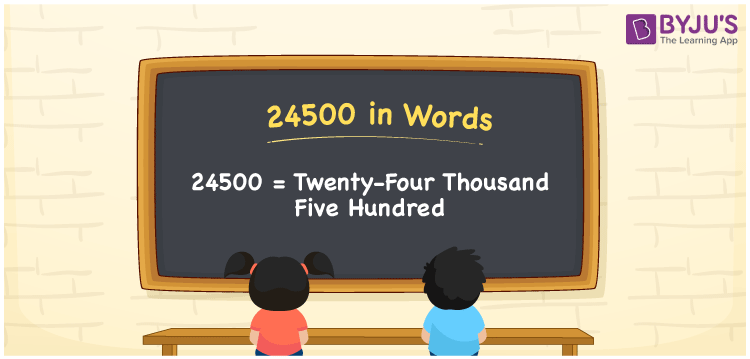# 24500 in Words

24500 in words is written as Twenty-four thousand five hundred. In both the International System of Numerals and the Indian System of Numerals, 24500 is written as Twenty-four thousand five hundred. The number 24500 is a Cardinal Number as it represents some quantity. For example, “that Smart-TV costs 24500 rupees”, 24500 describes the TV cost.

 24500 in words Twenty-four thousand five hundred Twenty-four thousand five hundred in number 24500

## 24500 in English Words

24500 in English words is read as “Twenty-four thousand five hundred.”## How to Write 24500 in Words?

To write 24500 in words, we shall use the place value chart. In the place value chart, put 2 in the ten thousands, 4 in the thousands, 5 in the hundreds, and 0 in the tens and the ones, respectively. Let us make a place value chart to write the number 24500 in words.

 Ten Thousands Thousands Hundreds Tens Ones 2 4 5 0 0

Thus, we can write the expanded form as

2 × Ten Thousand + 4 × Thousand + 5 × Hundred + 0 × Ten + 0 × One

= 2 × 10000 + 4 × 1000 + 5 × 100 + 0 × 10 + 0 × 1

= 20000 + 4000 + 500 + 0 + 0

= 24500

= Twenty-four thousand five hundred.

24500 is a natural number, the successor of 24499 and the predecessor of 24501.

24500 in words – Twenty-four thousand five hundred

• Is 24500 an odd number? – No
• Is 24500 an even number? – Yes
• Is 24500 a perfect square number? – No
• Is 24500 a perfect cube number? – No
• Is 24500 a prime number? – No
• Is 24500 a composite number? – Yes

## Frequently Asked Questions on 24500 in Words

Q1

### How to write 24500 in words?

24500 in words is written as Twenty-four thousand five hundred.
Q2

### How to write 24500 in words in the International and Indian System of Numerals?

In both, the system of numerals, 24500 in words, is written as Twenty-four thousand five hundred.
Q3

### How to write 24500 in a place value chart?

In the place value chart, write 2 in the ten thousands, 4 in the thousands, 5 in the hundreds, and 0 in the tens and the ones, respectively.